﻿ Adsorption Studies of Aqueous Solutions of Methyl Green for Halloysite Nanotubes: Kinetics, Isotherms, and Thermodynamic ParametersPublications are Open
Access in this journal
Article Versions
Export Article
• Normal Style
• MLA Style
• APA Style
• Chicago Style
Research Article
Open Access Peer-reviewed

### Adsorption Studies of Aqueous Solutions of Methyl Green for Halloysite Nanotubes: Kinetics, Isotherms, and Thermodynamic Parameters

Y. M. Vargas-Rodríguez , A. Obaya, J. E. García-Petronilo, G. I. Vargas-Rodríguez, A. Gómez-Cortés, G. Tavizón, J. A. Chávez-Carvayar
American Journal of Nanomaterials. 2021, 9(1), 1-11. DOI: 10.12691/ajn-9-1-1
Received February 19, 2021; Revised March 22, 2021; Accepted March 30, 2021

### Abstract

Halloysite nanotubes (HNTs) were used to successfully remove methyl green dye from water. The HNTs were also characterized by X-ray diffraction (XRD), scanning electron microscopy (SEM), transmission electron microscopy (TEM), 29Si and 27Al magic angle spinning nuclear magnetic resonance with magic angle spinning (MAS-NMR) and nitrogen adsorption at 77 K. SEM and TEM micrographs showed that HNTs have lengths of 0.2 to 1.5 μm, an outer diameter of 100 nm and lumen of 20 nm wide. X-ray diffraction patterns showed that the HNTs were totally dehydrated. HNTs may be regarded as a mesoporous material with a pore size distribution in the range of 1.5-150 Å and specific surface area of 34.49 m2•g-1. The adsorption kinetics and equilibrium data of the dye, initial dye concentration, temperature, pH and contact time effect on removal efficiency were also investigated. Pseudo-first-order, pseudo-second-order, intraparticle diffusion and Elrich models were evaluated in order to determine the rate parameters. The adsorption rate followed pseudo-second-order kinetic model. Adsorption revealed that methyl green was adsorbed as the Langmuir isotherm model describes and the maximum adsorption capacity of the HNTs was achieved (185 mg•g−1), being an efficient adsorbent for methyl green adsorption.

### 1. Introduction

Industries such as textile, leather, paper, plastics, and cosmetics use dyes in their processes, resulting in the generation of a considerable amount of colored wastewater 1. It is estimated that there are more than 100,000 commercially available dyes with over 700,000 t of dyestuff produced annually and around 10% of the dyes are discharged into effluent from textile and related industries 2. These effluents, that are often strongly colored, produce aesthetical and toxicological damages when they are disposed into water or soil 3.

Methyl green (MG) is used in medicine and biology 4, 5 MG is, in a basic medium, a divalent cation, but into acidic conditions, it is a monovalent cation 6.

There are several methods used in the wastewaters treatment, both conventional (including biological) 7, 8, 9, 10, advanced processes include chemical and photochemical oxidations, nanofiltration and adsorption 11, 12, 13. Adsorption processes have been widely used for color and odor removal from water. Among the adsorbents used for wastewater dye treatment, activated carbon the oldest adsorbent known, is still effective for the most of common organic water pollutants 14, 15, 16, 17. On the other hand, inorganic adsorbents like zeolites 18, 19, 20, 21, alumina 22, 23, 24, silica gel 25, 26 and clay minerals 27, 28, 29, 30 are effective and they can support oxidizing environments. Clay minerals are abundant, inexpensive, and generally safe for environmental applications. Due to their large specific surface area, high porosity, surface charge and surface functional groups, clay minerals function as adsorbents, flocculants, and filtration media 31. HNTs is an economically viable material for these water treatment goals. HNTs are chemically like kaolin and are laminar clay minerals composed of two layers, which are wounded into a tubular morphology. The outer layer is composed of silicates (SiO2) in a tetrahedral crystalline arrangement; the inner layer of the nanotube is formed by alumina (Al2O3) with an octahedral crystalline arrangement, arrangements that conforms a system known as halloysite nanotube (HNTs) 32. The size of natural halloysite particles varies between 1-15 micrometers in length, with an inner diameter of 10-150 nm, depending on the deposits. The HNTs in their natural state can present traces of calcium oxide, magnesium, manganese, sodium, potassium and iron 33. Since the active surfaces these HNTs have, they have been used as adsorbents to efficiently remove dyes, such as neutral red, methylene blue, malachite green, rhodamine and anionic chrome azurol, from aqueous effluents 34, 35, 36, 37.

MG adsorption in aqueous solution has been previously studied with materials like montmorillonite 38, sepiolite 6, 39 amberlite 40, graphene sheets 41, and reduced graphene oxide 42. In this work, a study of MG removal by adsorption on HNTs and the evaluation of the effects of initial concentration, temperature and contact time is presented. Also, a kinetic study was performed with intraparticle diffusion model, first-order, second-order and Elovich models. An equilibrium study was achieved by using Temkin, Freundlich, Langmuir and Dubinin-Radushkevich isotherms.

### 2. Materials and Methods

2.1. Raw Materials

HNTs and rade methyl green (analytical reagent g) were purchased from Sigma-Aldrich. Deionized water was obtained with a Millipore deionizer, with a conductivity of 5.1 mhos. MG stock solution was prepared with a concentration equal to 600 mg/L in deionized water. It was subsequently diluted to the required concentrations.

2.2. Methods
2.2.1. Characterization

Crystalline phase identification was carried out by XRD (Bruker AXS, D8 Advanced Plus X-ray diffractometer, with monochromatic CuKa1 radiation, l = 1.54056 Å). Data collection was performed over the scan range 2 to 70 2θ, with a step width of 0.02 and a count time per step of 1.8 sec. Normal operating conditions were 35 kV and 30 mA. SEM performed morphological characterization of samples, with a field emission JEOL JSM 7600F equipment, whose working conditions included a low accelerating voltage, 1 to 10 kV, and working distances from 4.7 to 7.6 mm. Micrographs were acquired with both backscattered and secondary electrons. This microscope was equipped with X-ray energy detector (X_ACT Oxford INCA) for chemical microanalyses (EDS or EDX). To complete the morphological characterization, studies were carried out by TEM (JEOL 1200 EX microscope, operating at 120 kV and 70 μA). 27Al and 29Si MASNMR spectra were recorded with a 300 MHz spectrometer (Bruker, Billerica, MA). A 4.5 µs pulse (corresponding to 90°) was used with a repetition time of 5 s between pulses to avoid saturation effects; the spinning frequency was in the range of 4.0-4.5 kHz; the minimum number of scans per spectral data collection was 500. NMR measurements were carried out at room temperature. The specific surface area of the samples was determined by the Brunauer-Emmett-Teller (BET) method. The pore volume and pore-size distribution was estimated by the Barrett-Joyner-Halenda (BJH) method at 77 K (Autosorb 1 MP, Quantachrome Instrument). Prior to measurements, samples were degassed under vacuum at 573 K for 10 h.

A spectrophotometric calibration curve was performed to determine the concentration of MG in water. Measurement wavelength is 632 nm. The concentrations were between 10 and 300 mg•L-1. A set of these solutions was called as system. Each system was kept under constant stirring at 600 rpm at different temperatures (297.15, 313.15 and 333.15 K). Then, 20 mg of HNTs were added to each of the systems at a different temperature. Then, the suspension was centrifuged at 2500 rpm for 3 minutes, to separate and recovers supernatant HNTs. The concentration of the dye before and after adsorption was determined using a spectrophotometer (Varian, Lambda 25). The amount of MG adsorbed by HNTs at any time was calculated from the concentration in the solution before and after adsorption; this amount was calculated from the mass balance equation, Eq. 1.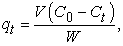(1)

qt is the amount of MG (mg•g-1) adsorbed by NTHS; C0 and Ct (mg•L-1) were the concentrations of MG before and after the contact time t, respectively; V (L) was the volume of the solution of MG and W (mg) the mass of the HNTs. Experimental procedures under equilibrium were identical to the kinetic tests. The amount of dye adsorbed at equilibrium, qe (mg•g-1), was calculated from the following equation.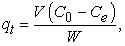(2)

Where, C0 and Ce (mg•L-1) were the liquid-phase concentrations of dye, initially and at equilibrium, respectively; V (L) is the volume of the solution and W (mg) is the mass of the adsorbent.

### 3. Results and Discussion

3.1. Characterization

X-ray diffraction results are shown in Figure 1. Peaks at 2θ values of 12.2°, 20.1°, 24.5°, 35.1°, 38.1°, 54.6° and 62.6°, indicate the presence of a HNTs. This identification can be remarked by two important results for the d001 reflection near to the 12.2° peak, which were observed in the pattern: this peak corresponds to a d-spacing around 7.2 Å a characteristic result for a dehydrated HNTs (7 Å) and the intensity of this peak is the half of that of the peak located at 20.1º, in 2θ scale 43. Similar XRD results have been observed for samples from New Zealand 33, 44, 45.

3.2. 27Al and 29Si MAS-NMR

The spectrum for 29Si, Figure 1b, showed a signal at -93.027 ppm. This signal is typical of a Q3(0Al) basic silicate structure, a layered-clays like kaolinite and a variable degree of ordering in the Si O Si subunits. 27Al MAS-NMR spectrum for HNTs shows a signal at 0.312 ppm, characteristic for an octahedral symmetry for Al, Figure 1c 46.

• Figure 1. a) XRD pattern for halloysite nanotubes (HNTs)
• Figure 2. SEM micrographs of halloysite nanotubes at different magnifications
3.3. SEM, TEM and EDS

The chemical composition of the HNTs in mass percentage were SiO2, 53.10; Al2O3, 39.05; CaO, 2.11; MgO, 0.02; K2O, 2.74; 0.45, 0.02; MnO2, 2.50. The SiO2 :Al2O3 ratio is similar to that which was found in the Algerian HNTs 47, 48 and that from Camerun 49. SEM micrographs of the HNTs, Figure 2, showed nanotubes agglomerates unevenly with lengths from 0.2 to 1.5 μm. The transmission electron micrograph, Figure 2d, showed an outer diameter of 100 nm and lumen of 20 nm. These dimensions agree with reported data 50. The chemical composition of the HNTs shows a SiO2:Al2O3 theoretical ratio like that of kaolinite with high water content 51.

• Figure 3. TEM micrographs of halloysite nanotubes (HNTs) studied

Analyses of adsorption and desorption of nitrogen were carried out to investigate the surface area and pore volume of HNTs, Figure 4a.The adsorption-desorption isotherm showed a tiny hysteresis cycle, H3 type, which closes to a relative pressure of approximately 0.42. The formation of this hysteresis cycle is characteristic of mesoporous materials (2-50 nm), which form agglomerates of particles with pores or slit-laminar shaped, and / or non-uniform size 52; the closing of the hysteresis loop is generated by the presence of particles with pore radius in the micropore and mesopore border (1.7-2.0 nm), which means that the Kelvin equation may not apply for that size of small pores i.e. < 2 nm which are equivalent to these relative pressures. Similar hysteresis loops were obtained for HNTs of New Zealand and Western Australia 53 and for a HNTs purchased from Sigma-Aldrich 54.

Figure 4b shows the pore size distribution of HNTs, obtained by the Barrett-Joyner-Halenda (BJH) method. The sample shows mesopores and macropores. The distribution of pore size has two maximum pore size, with mesopore diameters oft 3.7 and 9.3 nm, respectively. The concentration of fine pores, which has been observed for halloysite, has been attributed to the tubular shape of halloysite particles. The tubes provide the characteristic small pores directly, due to their hollow interiors, and also indirectly, due to the voids, which are created when they pack together 55.

Finally, the specific surface area was determined by the Brunauer-Emmett-Teller (SBET) method 56. The SBET for HNTs was 34.49 m2•g-1. A low value if compared to those obtained for natural HNTs from Algeria, New Zealand, Australia and Hunan (China), with 64, 58, 56 and 50.4 m2•g-1, respectively 47, 50, 53. However, the HNTs from Sigma-Aldrich had a similar SBET value, 32.76 m2•g-1 54, 57.

3.5. Removal of Methyl Green by HNTs: Adsorption Studies
3.5.1. Effects of Contact Time and Initial Dye Concentration

The kinetic profiles for adsorption of methyl green on HNTs were studied to determine the effect of initial concentrations of methyl green. Initial concentrations of the dye were 20, 40, 100 and 200 mg•L-1, as shown in Figure 5. It is observed that the rate of adsorption increased proportionally to the initial concentration. In addition, the adsorption of MG was faster along the first minutes and then reached a maximum, because the system approached to equilibrium conditions on adsorption.

• Figure 4. a) Adsorption and desorption of nitrogen for HNTs; b) Pore size distribution of halloysit, obtained by the BJH method
• Figure 5. a) Effect of contact time on adsorption of MG on HNTs from aqueous solutions (297.15 K, pH=6); b) Effect of initial dye concentration on adsorption of MG on HNTs from aqueous solutions (297.15 K, pH = 6); c) Effect of pH on the removal of MG on HNTs from aqueous solutions (297.15 K) and d) Effect of temperature in the removal rate of MG on HNTs from aqueous solutions, with C0= 200 mg/L and pH=6

It was observed that the maximum amount of MG adsorbed per gram of HNTs increased depending on the initial concentration of MG, from 4.95 to 77 mg, for the conditions of pH 6, 120 minutes and 24°C. Then, the percentage of adsorption for each system was determined, using Eq. 3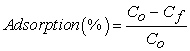(3)

Where C0 is the initial concentration and Cf is the final concentration (after 120 min) of MG. In Figure 5, there is the plot of adsorption percentage as a function of initial concentration; it indicates that when the concentrations of methyl green in aqueous solution on HNTs are very low, i.e., almost 100% of the initial concentration is adsorbed, most of the active sites of the particles of adsorbent are available, on the other hand if the MG concentration increases the adsorption is reduced because most of the active sites are saturated. It is important to observe that, in the concentration range studied, the results of adsorption vs C0 fitted a straight line, which intercepts with the 100 % point and has a slope = -0.1186 and r2 = 0.9926 (Figure 5).

3.5.2. Effect of pH

The effect of pH on the adsorption was studied in the pH range 2 and 10. There were evaluated 3 initial concentrations of MG: 20, 28 and 41 ppm, as presented also in Figure 5. With the results of initial and final concentrations the adsorption was determined, for each initial concentration. Two different tendencies were observed; at acidic conditions, the adsorption was increased proportionally with pH, up to 6; at basic environments (pH from 6 to 10), the adsorption of MG on HNTs remained constant. It might be explained because that, in this pH range, methyl green has carbinol, but has not dicationic species. These findings are like those reported for the VM adsorption in montmorillonite, where it has been shown that the carbinol is adsorbed in greater amounts than dicationic species of methyl green 37. Furthermore, with an increase in pH, the surfaces of HNTs have greater negative charge, increasing the electrostatic attraction between the cationic species of methyl green and the adsorption sites negatively charged, which increases the dye adsorption 33.

3.5.3. Effect of Temperature

The effect of temperature, on the adsorption of methyl green, was performed at 297.15, 313.15 and 333.15 K; the adsorbed amount of methyl green (MG) on the adsorbent mass vs time of adsorption was plotted. Results, shown in Figure 5, indicated that the dye adsorption process was favored at higher temperatures.

To interpret the experimental data on the rate of the adsorption of methyl green on HNTs, pseudo-first order, pseudo-second order, intraparticle diffusion and Elrich kinetic models were carried out.

3.6.1. Pseudo-first Order Kinetic Model

Pseudo-first order model is defined by Eq. 4 58: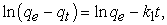(4)

where qe and qt are the values of the MG mass absorbed per unit mass (mg•g-1) at equilibrium and at time t, respectively, and k1 (min-1) is the pseudo-first-order adsorption rate constant. Then, ln (qe - qt) vs time was plotted, and data were fitted into a linear regression (Figure 6). With the values of the slope, the value of -k1 was obtained and, from interceptions with vertical axis, it was calculated the value of ln qe (for each temperature); these data are presented in Table 2.

3.6.2. Pseudo-second Order Kinetic Model

Pseudo-second order kinetic model 59 is expressed by Eq. 5:(5)

where qe is the mass absorbed per unit mass (mg•g-1) at equilibrium, qt is the amount of MG adsorbed per unit mass (mg•g-1) at any time and k2 (g•mg-1•min-1) is the pseudo-second order rate constant. To evaluate k2 in this model, t/qt was plotted as a function of time, Figure 6. After a linear regression, qe was determined from the inverse value of the slope and k2, from the intercept of the graph, Eq. (6). Results, which are presented in Table 2, indicate that the adsorption is faster as temperature increases.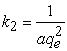(6)

3.6.3. Intraparticle Diffusion Kinetic Model

This model 60 is described by Eq. 7: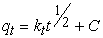(7)

where qt is the amount of the MG mass adsorbed per mass unit (mg•g-1) at time t, and ki (min-1/2) and C are constants. From a graph of the mass absorbed (qt) vs t1/2, Figure 6, data were fitted into a linear regression. From the slope, the intra-particle diffusion constants (ki) were obtained and, from interceptions with the vertical axis, the C values were determined. Results, which are presented in Table 2, show that the correlation parameters r2 ranged between 0.8 and 0.9; thus, data did not fit the model of intra-particle diffusion.

3.6.4. Elovich Kinetic Model

Roginsky and Zeldovich proposed an equation 61 known as the Elovich model, Eq. (8), which considers that the rate-controlling step factor, parameter is the diffusion of the dye molecules and chemisorption kinetics 62, this model has been successfully applied to systems in which the adsorption surface is heterogeneous.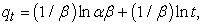(8)

where qt is the adsorbed amount of MG per unit mass of adsorbent (mg•g-1) at any time t (min), α is the initial adsorption rate and β is the Elovich coefficient related to the extent of surface coverage and activation energy. A graph qt vs ln t was plotted, Figure 6, and then it was fitted by a linear regression. The parameter α was obtained from the intercept (a) and slope (b) of Eq. (9) and the term β, from the inverse value of the slope.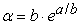(9)

Results, that are plotted in Figure 6 and summarized in Table 2, indicate that the correlation coefficients, for the three systems at different temperatures, were lower than 0.972, which indicates that the adsorption of methyl green on HNTs is not a chemisorption process.

• Figure 6. Model for kinetics adsorption of MG on HNTs: a) Pseudo-first order (pH = 6.0 and Co= 200 ppm); b) Elovich (pH = 6.0 and Co = 200 ppm); c) Intra-particle (pH = 6.0 and Co = 200 ppm), and d) Pseudo-second order (pH = 6.0 and T = 297.15 K).

The best correlation for these results was for the pseudo-second order model and it indicates that the rate of adsorption of methyl green on HNTs increased with temperature.

3.6.5. Activation Energy

To determine the activation energy for the adsorption of methyl green on HNTs, between 297.15 and 333.15 K, it was used the linear form of Arrhenius equation (Eq. 10):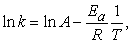(10)

where k is the constant of pseudo-second order rate (min-1•mg-1•g), A is the frequency factor (min-1 mg-1•g•min), Ea is the activation energy (J/mol), R is the universal gas constant (8.314 J•mol-1•K-1) and T is the temperature (K). Thus, from the graph ln k as a function of reciprocal temperature, a straight line with slope equal to (Ea/R) and intercept (ln A) was obtained, Figure 7a. With the slope value, Ea resulted to be 31 547 J•mol-1.

To describe the behavior of equilibrium adsorption of methyl green on HNTs, data of the adsorbed amount of methyl green per gram of adsorbent, at equilibrium, were obtained at 297.15, 303.15 and 313.15 K, Figure 7b. An increase of the adsorbed amount of methyl green on HNTs, according to the equilibrium concentration, was observed. It is because at higher concentrations of the dye, it is greater the shift of the adsorption-desorption equilibrium towards the adsorption process until reaching the highest concentrations, which shows a saturation of the active sites of the adsorbent. Therefore, the values r2 of qe increased as the methyl green equilibrium concentrations increased. Results, at equilibrium, were assessed by fitting data into Temkin, Freundlich, Langmuir and Dubinin-Radushkevich (DRK) adsorption isotherms.

3.7.1. Temkin Isotherm

The Temkin model 62 is linearly represented by equations (11) and (12). There qe is the amount of adsorbed methyl green (mg•g-1) at equilibrium, At is Temkin isotherm equilibrium binding constant (L•g-1) and B is a constant related to heat of sorption (J mol-1), Ce is the concentration of the dissolution of methyl green (mg•L-1) at equilibrium, bT is the Temkin isotherm constant (dimensionless) and R the universal gas constant (8.314 J•mol-1•K-1).(11)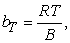(12)

Then, a plot of qe as a function of ln Ce (Figure 8a) allows, by fitting these data into a linear regression, to determine B from the slope and At from the intercept (a), using Eq. 13.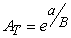(13)
• Figure 7. Arrhenius plot for adsorption of methyl green on HNTs, at different temperatures with pH=6.0 and C0 = 200 ppm (a) and Adsorption isotherms of methyl green on HNTs at pH = 6.0 (b)

The linear regression parameters r2 for the three operating temperatures are above 0.99 indicating that these results fitted well the Temkin model, probably because this model is valid for solute concentrations that are not close to zero, or at high concentrations, like some of the experiments carried out in this work (Table 2). According to the model, the adsorption of methyl green on HNTs is characterized by a uniform distribution of the binding energy and heat of adsorption (as a function of temperature) of all the molecules in the layer, which decreases linearly with coverage 63.

3.7.2. Freundlich Isotherm

The Freundlich 64 adsorption isotherm is commonly used to describe the adsorption processes on heterogeneous surfaces, and it is mathematically expressed by Eq. 14.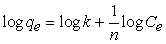(14)

where Ce (mg•L-1) is the equilibrium concentration of methyl green, qe (mg•g-1) is the equilibrium amount of adsorbed methyl green, k (mg•g-1) and n are constants for a given adsorbate and adsorbent, per mass unit of adsorbent, at equilibrium. High values of k indicate an easy uptake of the adsorbate. The slope 1/n of Freundlich equation indicates the heterogeneity of the surface of the adsorbent, where values of 1/n close to zero imply higher heterogeneity whilst values of 1/n closer to unity imply a lower heterogeneity.

To evaluate the results by the Freundlich isotherm, a graph log qe vs log Ce was plotted at 297.15, 313.15 and 333.15 K, Figure 8b. Data were fitted into linear regressions; with the inverse value of the slope the value of n was obtained and K with the intercept. These results are presented in Table 3.

3.7.3. Langmuir isotherm

The Langmuir isotherm 65, mathematically shown in Eq. 15, assumes a monolayer adsorption.(15)

where Ce (mg•L-1) is the equilibrium concentration, qe (mg•g-1) is the amount of adsorbate, which was adsorbed per unit mass of adsorbent and q0 and KL are the Langmuir constants related to the adsorption capacity and the rate of adsorption, respectively. From a graph of Ce / qe vs Ce, q is obtained from the slope and KL from the intercept, Figure 8c. Results are presented in Table 3.

Data were fitted into the Dubinin-Radushkevich (D-R) adsorption isotherm, in its linear form. This model is generally applied to express the adsorption mechanism with a Gaussian energy distribution on a heterogeneous surface 66.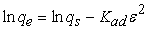(16)

where qe is amount of adsorbate in the adsorbent (mg•g-1) at equilibrium, qs the theoretical isotherm saturation capacity (mg•g-1), Kad the Dubinin-Radushkevich isotherm constant (mol2•K-1•J-2) and ε the Rubinin-Radushkevich isotherm constant and is calculated using Eq. 17.(17)

where R is the gas constant, 8.314 J•mol-1•K-1, and T the absolute temperature (K). The graph ln vs ε2 is shown in Figure 8d. The results are presented in Table 2, it is observed that for the three temperatures R2.

• Figure 8. Adsorption isotherms for methyl green dye adsorption on HNTs, at different temperatures: a) Temkin: b) Freundlich isotherms; c) Langmuir and Dubinin-Raduskevich

Table 3 shows that the obtained results, for the three experimental temperatures, fitted Temkin and Langmuir equations, with r2 of 0.9962, 0.9967 and 0.9994 for the Temkin equation and r2 of 0.9982, 0.9986 and 0.9994 for Langmuir equation. The results fitted to Temkin equation possibly due to the concentration range studied (neither very close to zero nor high concentrations). However, the correlation to the Langmuir equation is slightly higher.

The maximum amount of methyl green that the HNTs adsorb, at 273.15 K, is 187.18 mg on g of HNTs. Table 4 shows the results of maximum adsorption of methyl green on different adsorbents, the studies were carried out both in the Batch system and in Fixed bed adsorption. It is observed that the adsorbents with the highest adsorption capacity for methyl green are sepiolite phyllosilicates and HNTs with 65.32 and 187.18 mg g-1 respectively. The result of this study is due to the fact that the structure of the nanotubes has a lumen in addition to an external surface, so the methyl green was mainly encapsulated in the lumen and was partially charged on the surface, so that the HNTs functioned as carriers of the dye, in addition to being adsorbent, as has happened with other compounds such as ibuprofen 67, and dyes such as methylene blue 33, red neutral 32, Malachite green 34 and Methyl violet 68. These results indicate that HNTS are an efficient adsorbent for methyl green.

3.7.5. Thermodynamic Parameters

The constant adsorption for Langmuir isotherms (KL) at 297.15, 313.15 and 333.15 K was used to determine thermodynamic parameters: the variation in the standard enthalpy (ΔH°) and standard entropy (ΔS°), by using Eq. 18.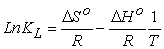(18)

where KL is Langmuir constant when concentration terms are expressed in L•mol−1, R is the universal gas constant (8.314 J•mol−1•K−1) and T (K) is the temperature. The ΔH° and ΔS° values can be calculated from the slope and intercept of the plot of Ln KL vs 1/T (Figure 9). The standard Gibbs free energy changes (ΔG°) at temperatures of 297.15, 313.15 and 333.15 K, was determined using Eq. 19.(19)
• Figure 9. Ln constant adsorption for Langmuir isotherms vs 1/T plot for adsorption of methyl green on HNTs

The changes in the activation energy (Ea) that are presented in Table 5 can be interpreted as follows: if they are low (5 to 40 kJ mol−1), the main process is a physisorption and, if they are high (40-800 kJ•mol−1), main process is chemisorption 65. The activation energy for MG adsorption on HNTs resulted to be 31.45 kJ•mol−1, showing that physisorption is the principal process. The Gibbs free energy values were negative, which indicates that the adsorption is spontaneous. The decrease in the value standard free energy with increasing temperature reveals that the adsorption of MG in HNTs becomes more favorable at higher temperature. The positive value of ΔH0 also confirms that the adsorption is endothermic. The positive value of ΔS0 inferred increased randomness MG during adsorption in active sites of HNTs 37.

### 4. Conclusions

A kinetic and thermodynamic study of the adsorption-adsorption of methyl green on HNTs was carried out, finding that the nanotubes used have lengths from 0.2 to 1.5 μm, an outer diameter of 100 nm and lumen of 20 nm. The effect of time and concentration on the adsorption of MG was studied, after 10 minutes the adsorption is practically independent of time, the% of adsorption is inversely proportional to the initial concentration. The optimal adsorption pH for MG is between 6 and 7. It was found that the adsorption kinetics of methyl green on HNTs fitted properly to a pseudo- second order kinetics model.

The adsorption isotherms were adjusted to the Langmuir equation, indicating that the adsorption is localized, only at defined (active) sites on the surface, that the surface of the HNTs is homogeneous and a methyl green monolayer is formed, if each adsorption site can adhere only one adsorbate molecule, and that there is no interaction between the adsorbed molecules, and the maximum amount that 1 g of HNTs can adsorb is 187. 7mg L-1, a result much higher than those reported in other studies on the adsorption of methyl green with other adsorbents.

The increase in temperature in the adsorption induces a slight increase in the adsorption of MG and considering that the adsorption isotherms conform to the Langmuir equation, this energy is the same for all active adsorption sites., The value small the activation energy 31.45 (kJmol −1), indicated that the adsorption of methyl green was a physisorption process, and the negative values of ΔG° (kJmol − 1), indicate that the process is spontaneous.

Because of HNTs occur naturally, they are inexpensive and can be used as mined without further treatment, under the mild adsorption conditions of methyl green (room temperature and pH: 6-7), because the process is spontaneous, because the adsorption is physical, since the methyl green was encapsulated in the lumen and was partially charged on the surface, so that the HNTs function as carriers of the methyl green, Finally, the HNTs are an efficient adsorbent for the adsorption and recovery of methyl green in aqueous medium.

### Acknowledgements

The authors thank Sofia Gallardo-González for technical assistance. This work was supported by UNAM-PAPIIT IN229119 and PIAPI2056.

### References

Published with license by Science and Education Publishing, Copyright © 2021 Y. M. Vargas-Rodríguez, A. Obaya, J. E. García-Petronilo, G. I. Vargas-Rodríguez, A. Gómez-Cortés, G. Tavizón and J. A. Chávez-CarvayarThis work is licensed under a Creative Commons Attribution 4.0 International License. To view a copy of this license, visit http://creativecommons.org/licenses/by/4.0/